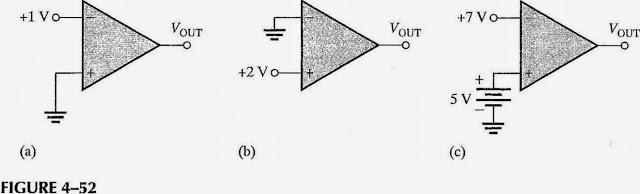# Confusion about open-loop op-amp comparator operation

Gold Member

## Homework Statement

Determine the output level (maximum positive or maximum negative) for each comparator in Figure 4-52.## Homework Equations

$$A_{ol}=\frac{V_{out}}{V_{in}}$$

## The Attempt at a Solution

I am new to comparators but I thought that since all of these op-amps are open-loop configurations, the output voltages would be pinged at ±Vmax where ±Vmax is the maximum output voltage of the op-amp according to its datasheet.

So, for (a), my answer was:

$$V_{out(max)}=(A_{v(ol)})(V_{in(max)})=(A_{v(ol)})(-1V)$$

However, I was told "you don't need the open-loop gain of the amplifier. You need to compare the voltages in the noninverting input to the voltages in the inverting input, then decide the output of the comparator."

Still... I know that even a small difference between the two inputs of an op-amp without feedback can drive the device into saturation and thus the output voltage to ±Vmax.

How do I rectify what I believe to be true about comparators with what I was told by my professor?

berkeman
Mentor
Hey JJ!

Yeah, if the inputs are different by hardly anything, the output will be pinned at (or near) the supply rails. To know how close, you would need specs on the comparators themselves. In fact many comparators have an open collector output with respect to ground, which doesn't seem to be reflected in the diagram that you are given.

So my guess is that you are just supposed to say which rail the output is pinned at for each of the 3 configurations...?

Gold Member
...many comparators have an open collector output with respect to ground, which doesn't seem to be reflected in the diagram that you are given.

So my guess is that you are just supposed to say which rail the output is pinned at for each of the 3 configurations...?

Hi berkeman. Thanks again for your input. I'm nearing the end of my B.S. and I sort of took this op-amps course out of order. Going from control systems to this is a backwards transition to say the least.

I think what the professor is looking for in terms of the "correct" answer is (a) -Vout(max), (b) +Vout(max) and (c) -Vout(max).

Too bad I can't see inside the black box that is the op-amp. Is internal op-amp design a Master's level course or can I find it somewhere at the B.S. level? The other thing is that we linearize everything. Heaven forbid we would have messy integrals to deal with!

berkeman
Mentor